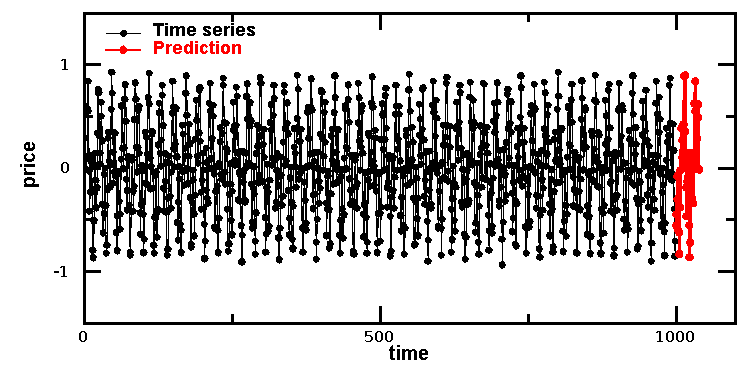# DMelt:Finance/Market Prediction

Limitted access. First login to DataMelt if you are a full DataMelt member. Then login to HandWiki as a user.

# Market prediction

In Section Neural Network we discussed how to build a neural net based on backpropogation algorithm. Now we will show how to use this neural network approach to make predictions of financial market market.

# Using Joone

Let us consider Joone Neural net for forecasting market predictions using a generated time series in the form cos(x)*sin(x)+Gaussian noise.

Here is the output image that shows the original time series and the predicted trends (n red).As you can see, the neural net correctly predicts the expected behavior.

# Market prediction

Now we consider another practical example. First, we will fetch data from Yahoo and store this data in a file: YahooFinance.egb. We will gather training data for the last 2 years, stopping 60 days short of today.

This script also prints input (10 values) and output (one value): A single entry out of 491 will look as this:

[0.00384, 0.00178, 0.006807,
-0.00833, 0.00795, 0.014900,
-0.00409, 0.00220, -0.00312,
-0.00934]
ideal= [-0.01494]

Now let us build a neural net with 10 inputs, one output and 20 hidden layers. We will train this network for 1 minute and then save the trained network in a file. The script code is given below. <ifauthr>

</ifauth>

Now we read data from YahooFinance again, this time using last 30 days. Then we read trained neural network and perform the prediction based on the last 2 year trend. We compare the actual and predicted value, and print likelihood of our correct prediction:

The output of the execution of this script will look something like:

Fetching data from Yahoo ..
Print prediction..
Day  1.0 :actual= -0.0113 ( -1 ) predict=-0.0041 ( -1 ),diff= 0.00723281045594
Day  2.0 :actual= -0.0108 ( -1 ) predict=0.0056 ( 1 ),diff= 0.016323498302
Day  3.0 :actual= 0.0070 ( 1 ) predict=0.0068 ( 1 ),diff= 0.000142392841989
Day  4.0 :actual= 0.0320 ( 1 ) predict=0.0026 ( 1 ),diff= 0.0294366145542
Day  5.0 :actual= 0.0226 ( 1 ) predict=-0.0030 ( -1 ),diff= 0.0256697221103
Day  6.0 :actual= 0.0055 ( 1 ) predict=0.0132 ( 1 ),diff= 0.00767318237177
Day  7.0 :actual= -0.0080 ( -1 ) predict=0.0118 ( 1 ),diff= 0.0198059904802
Day  8.0 :actual= -0.0057 ( -1 ) predict=0.0092 ( 1 ),diff= 0.0148906339578
Day  9.0 :actual= 0.0176 ( 1 ) predict=0.0020 ( 1 ),diff= 0.0156139264763
Day  10.0 :actual= -0.0038 ( -1 ) predict=0.0031 ( 1 ),diff= 0.00693045712649
Day  11.0 :actual= 0.0156 ( 1 ) predict=-0.0033 ( -1 ),diff= 0.0188739030904
Day  12.0 :actual= -0.0018 ( -1 ) predict=0.0065 ( 1 ),diff= 0.00835930166595
Day  13.0 :actual= 0.0022 ( 1 ) predict=0.0024 ( 1 ),diff= 0.000244326149596
Day  14.0 :actual= 0.0065 ( 1 ) predict=-0.0063 ( -1 ),diff= 0.0127469148732
Day  15.0 :actual= 0.0003 ( 1 ) predict=0.0071 ( 1 ),diff= 0.00687984465776
Day  16.0 :actual= 0.0034 ( 1 ) predict=-0.0029 ( -1 ),diff= 0.00624265349531
Day  17.0 :actual= -0.0158 ( -1 ) predict=0.0020 ( 1 ),diff= 0.0177893230083
Day  18.0 :actual= 0.0032 ( 1 ) predict=-0.0046 ( -1 ),diff= 0.00781173668604
Day  19.0 :actual= -0.0172 ( -1 ) predict=0.0009 ( 1 ),diff= 0.0180277119123
Day  20.0 :actual= 0.0514 ( 1 ) predict=-0.0004 ( -1 ),diff= 0.0518078026793
Day  21.0 :actual= -0.0046 ( -1 ) predict=0.0060 ( 1 ),diff= 0.010602114572
Day  22.0 :actual= 0.0057 ( 1 ) predict=0.0051 ( 1 ),diff= 0.000595736696516
Day  23.0 :actual= 0.0154 ( 1 ) predict=0.0064 ( 1 ),diff= 0.00904954951323
Day  24.0 :actual= 0.0124 ( 1 ) predict=0.0152 ( 1 ),diff= 0.00284543760372
Day  25.0 :actual= -0.0018 ( -1 ) predict=-0.0040 ( -1 ),diff=
0.00223833948343
Day  26.0 :actual= 0.0092 ( 1 ) predict=0.0017 ( 1 ),diff= 0.0074896027783
Day  27.0 :actual= 0.0129 ( 1 ) predict=0.0012 ( 1 ),diff= 0.0116513741193
Day  28.0 :actual= 0.0149 ( 1 ) predict=0.0018 ( 1 ),diff= 0.0131001498602
Day  29.0 :actual= -0.0089 ( -1 ) predict=0.0062 ( 1 ),diff= 0.0151645127572
Day  30.0 :actual= -0.0006 ( -1 ) predict=-0.0028 ( -1 ),diff=
0.00226212010508
Day  31.0 :actual= -0.0020 ( -1 ) predict=-0.0015 ( -1 ),diff=
0.000477980160665
Day  32.0 :actual= -0.0142 ( -1 ) predict=-0.0026 ( -1 ),diff= 0.0116349624231
Direction correct: 25.0 / 32.0
Directional Accuracy: 63.1250 %